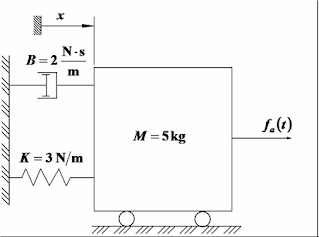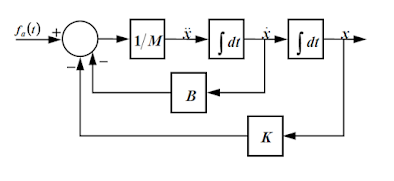## Welcome to the World of Modelling and Simulation

### What is Modelling?

This blog is all about system dynamics modelling and simulation applied in the engineering field, especially mechanical, electrical, and ...### Modelling a Basic Second Order System in SIMULINK

Problem: Consider the mechanical system shown in the figure below. The system is at rest for t < 0. At t = 0 an impulseforce of 8 N is applied to the mass. The impulse lasts 0.06 seconds. The displacement x is measured from the equilibrium position just before the mass is hit by the impulse force. Find the mathematical model of the system, draw a block diagram to represent it, and plot the displacement and velocity of the mass during the first 25 seconds.Figure 1: Diagram of the System.

Solution: The mathematical model is given by the following equation:The block diagram for the system is given below:Figure 2: Block Diagram.Figure 3: Simulink Block Diagram.

The output variables (i.e., displacement and velocity of the mass) displayed by the scope is shown in the figure below.

After examining the SIMULINK model, you can see that blocks from the following libraries have been used:
• Sources library: the Pulse Generator block (the Clock block was not used because it was not requested to send any variable to the MATLAB Workspace)
• Sinks library: The Scope block
• Math Operations library: The Sum and Gain blocks
• Continuous library: The Integrator block
• Signal Routing library: The Mux blockFigure 4: Response Curve.

You should have noticed that two of the gain blocks (damper and spring) have been rotated 180 degrees with respect to its default orientation. This has been done by right clicking on the corresponding block and then selecting flip block from the format menu. In this case the applied load was modeled using the Pulse Generator block. The dialog box containing the parameters for this block is shown below.